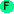# Math operations

## Math operations

The math operations of Arc are fairly limited. Many expected operations, such as trigonometry, are missing.* args Multiplication. ```>(* 2 3) 6 ```+ args Addition. This operator also performs string and list concatenation. ```>(+ 1 2 3) 6 ``` ```>(+ "ab" "c" "de") "abcde" ``` ```>(+ '(1 2) '(3 4) '(5)) (1 2 3 4 5) ```- args Subtraction. ```>(- 3 2) 1 ```/ args Division ```>(/ 1 2) 1/2 ``` ```>(/ 1.0 2) 0.5 ```cos arg Cosine function. New in arc3. ```>(cos 3.14) -0.9999987317275395 ```expt base exponent Exponentiation. ```>(expt 2 3) 8 ```log arg Natuarl logarithm function. New in arc3. ```>(log 100) 4.605170185988092 ```mod dividend divisor ```>(mod 10 3) 1 ``` ```>(mod -10 3) 2 ```rand [max] Returns a random number between 0 and 1, or between 0 and the specified integer (excluding the integer). ```>(rand 10) 5 ``` ```>(rand) 0.08773957245280758 ```sin arg Sine function. New in arc3. ```>(sin 3.14) 0.0015926529164868282 ```sqrt number Square root operation ```>(sqrt 2) 1.4142135623730951 ``` ```>(sqrt -1) 0+1i ```tan arg Tangent function. New in arc3. ```>(tan 3.14) -0.001592654936407223 ```trunc number Truncates to an integer. Was 'truncate' in arc0. ```>(trunc 1.9) 1 ``` ```>(trunc -1.1) -1 ```number n Tests if n is a number (int or num). ```>(number 3.14) t ```positive x Tests if x is a number and is positive (>= 0). This fails on complex numbers. ```>(positive 0) nil ``` ```>(positive -2) nil ``` ```>(positive "a") nil ```abs n Returns the absolute value. ```>(abs -3) 3 ```round n Rounds n to the nearest value. Halfs are rounded to the nearest even number. ```>(round 1/2) 0 ``` ```>(round 1.5) 2 ``` ```>(round 0.4) 0 ``` ```>(round -1.5) -2 ```roundup n Rounds n to the nearest value. Halfs are rounded away from zero. ```>(roundup 1/2) 1 ``` ```>(roundup 1.5) 2 ``` ```>(roundup 0.4) 0 ``` ```>(roundup -1.5) -2 ```nearest n quantum Rounds down to the nearest multiple of n. Renamed in arc3. ```>(nearest 3.14 0.1) 3.1 ``` ```>(nearest -5 10) -10 ```median list Returns the median of the list (the element at the midpoint of the list when sorted highest-to-lowest). Takes the earlier element for an even-length list. ```>(median '(1 5 3 2 4)) 3 ``` ```>(median '("dog" "cat" "bird") (median '(1 2 3 4))) Error: median: arity mismatch; the expected number of argu ments does not match the given number expected: 1 given: 2 arguments...: '("dog" "cat" "bird" . nil) 2 ```med list [compare-fn] Returns the median of the list (the element at the midpoint of the list when sorted according to compare-fn). Takes the later element for an even-length list. New in arc3. ```>(med '(1 5 3 2 4)) 3 ``` ```>(med '("dog" "cat" "bird") (med '(1 2 3 4))) Error: Function call on inappropriate object 2 ("bird" "cat" ) ```avg numbers Computes the average of a list of numbers. ```>(avg '(1+2i 0.4)) 0.7+1.0i ```even n Tests if n is even. Argument n must be an integer. ```>(even 1) nil ``` ```>(even 2) t ```odd n Tests if n is odd. Argument n must be an integer. ```>(odd 1) t ``` ```>(odd 2) nil ```max [arg ...] Returns the maximum arg. The args must be comparable with >. ```>(max 10 -3 5) 10 ``` ```>(max "cat" "dog" "bird") "dog" ```min [arg ...] Returns the minimum arg. The args must be comparable with <. ```>(min 10 -3 5) -3 ``` ```>(min "cat" "dog" "bird") "bird" ```multiple x base Tests if `x` is a multiple of `base`. ```>(multiple 10 5) t ``` ```>(multiple 11 5) nil ```

Copyright 2008 Ken Shirriff.